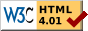## Dokumentation zu: next_bit(E)``` SYNOPSIS int next_bit (string str, int start) int next_bit (string str, int start, int find_cleared) DESCRIPTION Return the number of the next bit in bitstring after position . Usually this is the next set bit, but if is given and not 0, the position of the next cleared bit is returned. Note that finding cleared bits after the last set bit is limited to the actual length of . Each character contains 6 bits. So you can store a value between 0 and 63 in one character (2^6=64). Starting character is the blank " " which has the value 0. The first character in the string is the one with the lowest bits (0-5). EXAMPLES string s; int p; s = set_bit("", 4); s = set_bit(s, 2); for (p = -1; -1 != (p = next_bit(s, p); ) write(p+"\n"); --> will write 2 and 4 SEE ALSO set_bit(E), clear_bit(E), test_bit(E), last_bit(E), count_bits(E), and_bits(E), or_bits(E), xor_bits(E), invert_bits(E), copy_bits(E) ``` Die Seite ist auch in Deutsch vorhanden.

Start » Magierhandbuch » Docu » Efun » Next_bit Letzte Generierung: 25.04.2021, 01:58mud@wl.mud.de